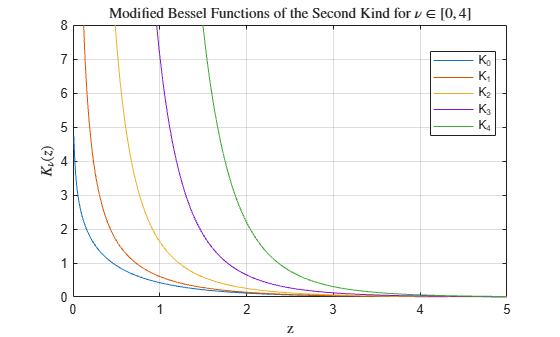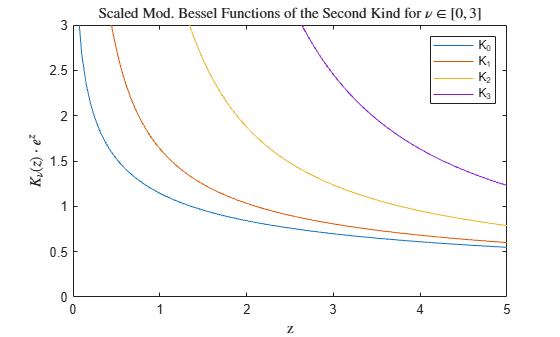# besselk

Modified Bessel function of second kind

## Syntax

K = besselk(nu,Z)
K = besselk(nu,Z,scale)

## Description

example

K = besselk(nu,Z) computes the modified Bessel function of the second kind Kν(z) for each element in array Z.

example

K = besselk(nu,Z,scale) specifies whether to exponentially scale the modified Bessel function of the second kind to avoid underflow or loss of accuracy. If scale is 1, then the output of besselk is scaled by a factor exp(Z).

## Examples

collapse all

Define the domain.

z = 0:0.01:5;

Calculate the first five modified Bessel functions of the second kind. Each row of K contains the values of one order of the function evaluated at the points in z.

K = zeros(5,501); for i = 0:4 K(i+1,:) = besselk(i,z); end

Plot all of the functions in the same figure.

plot(z,K) axis([0 5 0 8]) grid on legend('K_0','K_1','K_2','K_3','K_4','Location','Best') title('Modified Bessel Functions of the Second Kind for $\nu \in [0,4]$','interpreter','latex') xlabel('z','interpreter','latex') ylabel('$K_\nu(z)$','interpreter','latex')Calculate the scaled modified Bessel functions of the second kind ${\mathit{K}}_{\nu }\left(\mathit{z}\right)\cdot {\mathit{e}}^{\text{\hspace{0.17em}}\mathit{z}}$ for values of $\mathit{z}$ in the interval $\left[0,5\right]$ and for orders of $\nu$ between 0 and 3.

z = linspace(0,5); scale = 1; Ks = zeros(4,100); for nu = 0:3 Ks(nu+1,:) = besselk(nu,z,scale); end

Plot all of the functions in the same figure. For large values of $\mathit{z}$, the scaled functions do not underflow the limits of double precision as quickly as the unscaled functions, extending their range of computability.

plot(z,Ks) ylim([0 3]) legend('K_0','K_1','K_2','K_3') title('Scaled Mod. Bessel Functions of the Second Kind for $\nu \in \left[0, 3 \right]$','interpreter','latex') xlabel('z','interpreter','latex') ylabel('$K_\nu(z) \cdot e^{z}$','interpreter','latex')## Input Arguments

collapse all

Equation order, specified as a scalar, vector, matrix, or multidimensional array. nu is a real number that specifies the order of the modified Bessel function of the second kind. nu and Z must be the same size, or one of them can be scalar.

Example: besselk(3,Z)

Data Types: single | double

Functional domain, specified as a scalar, vector, matrix, or multidimensional array. besselk is real-valued where Z is positive. nu and Z must be the same size, or one of them can be scalar.

Example: besselk(nu,0:3)

Data Types: single | double
Complex Number Support: Yes

Toggle to scale function, specified as one of these values:

• 0 (default) — No scaling

• 1 — Scale the output of besselk by exp(Z)

The value of besselk decreases rapidly as the value of Z increases, so exponentially scaling the output is useful for large values of Z where the results otherwise quickly lose accuracy or underflow the limits of double precision.

Example: besselk(nu,Z,1)

collapse all

### Modified Bessel Functions

This differential equation, where ν is a real constant, is called the modified Bessel's equation:

${z}^{2}\frac{{d}^{2}y}{d{z}^{2}}+z\frac{dy}{dz}-\left({z}^{2}+{\nu }^{2}\right)y=0.$

Its solutions are known as modified Bessel functions.

The modified Bessel functions of the first kind, denoted Iν(z) and Iν(z), form a fundamental set of solutions of the modified Bessel's equation. Iν(z) is defined by

${I}_{\nu }\left(z\right)={\left(\frac{z}{2}\right)}^{\nu }\sum _{\left(k=0\right)}^{\infty }\frac{{\left(\frac{{z}^{2}}{4}\right)}^{k}}{k!\Gamma \left(\nu +k+1\right)}.$

You can compute the modified Bessel functions of the first kind using besseli.

The modified Bessel functions of the second kind, denoted Kν(z), form a second solution independent of Iν(z) given by

${K}_{\nu }\left(z\right)=\left(\frac{\pi }{2}\right)\frac{{I}_{-\nu }\left(z\right)-{I}_{\nu }\left(z\right)}{\mathrm{sin}\left(\nu \pi \right)}\text{\hspace{0.17em}}.$

## Version History

Introduced before R2006a